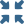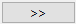# Create Envelope of Results

Identify the most significant result out of all selected analyses.

Before you begin, you must have one or more structural linear or multi-loadcase analysis results in the design study.

Envelope can be either max, min, or extreme. Envelope is mathemetically represented by the following equation for each point:
$\left\{{R}_{s}\right\}\text{\hspace{0.17em}}=\text{\hspace{0.17em}}\left(\text{min/max/extreme}\right)\text{\hspace{0.17em}}\left\{{c}_{1}\left\{{R}_{1}\right\},\text{\hspace{0.17em}}{c}_{2}\left\{{R}_{2}\right\},\text{\hspace{0.17em}}\dots \text{\hspace{0.17em}}{c}_{i}\left\{{R}_{i}\right\}\right\}$
Where,
${R}_{i}$
A vector of results (displacements, stresses, strains, and so on) for each individual load case.
${c}_{i}$
Scale factor.
min
Finds the minimum value among all the selected analyses weighted by appropriate scale factors.
max
Finds the maximum value among all the selected analyses weighted by appropriate scale factors.
extreme
Finds absolute maximum value among all the selected analyses weighted by appropriate scale factors. The sign of the number is not changed during result display. For example, extreme of 5 and -10 is -10.
1. On the main toolbar, select(Post study).
2. Select Structural envelope.
3. From the drop-down menu, select the type of envelope analysis.
4. In the dialog, hold down Control + Shift and select the results to combine.
5. Click thebutton to combine analyses.
6. Click Solve.
Structural envelope supports Results plot and Pick info post processing options.